# Parallelization of QuantumATK calculations¶

ATK uses a range of parallelization techniques to distribute computational workload over a number of computing cores (CPUs). This may either reduce the total wall-clock time or reduce the per-core peak memory requirement. We will here explore the basics of how to parallelize QuantumATK calculations, both for bulk systems and for NEGF device calculations.

ATK offers multi-level parallelization and will by default try to distribute the computing workload in the best way possible. However, manual tuning of parallelization settings may sometimes be beneficial. In this case, the QuantumATK user needs to be aware of an important distinction between two very different ways to “measure” the effect of parallelization:

Efficiency: If efficiency is the goal of a particular parallelization scheme (which it often is), the speed-up per extra CPU should be as high as possible. That is, a calculation that takes 1 hour in serial (on a single CPU) should ideally take 15 minutes on 4 CPUs. Parallelization efficiency is therefore important if efficient use of CPU resources is more important than the wall-clock time spent on the calculation. If the goal of parallelizing over several CPUs is to get the calculation to finish as fast as possible, one will usually choose to compromise on efficiency. For example, parallelizing a bulk calculation with 4 k-points over 40 CPUs will rarely give a speed-up of 40 compared to running the calculation on 1 CPU. However, it will in most cases still lead to a significant reduction of the wall-clock time, by a factor of maybe 10-20 depending on the system. This may therefore result in a less efficient parallelization scheme, when comparing the total CPU-time to the wall-clock time, but may be preferred if getting the result quickly is more important than very efficient use of computing resources.## Unit-of-work¶

When distributing an entire QuantumATK calculation over a number of CPUs, the smallest “package” of computational workload that is conveniently assigned to a single CPU is one “unit-of-work”. Importantly, this entity is not the same for bulk and device (NEGF) calculations.

Bulks Devices (NEGF)
1 k-point 1 contour point

For a bulk calculation, the natural unit-of-work is one single k-point: A bulk calculation with 4 irreducible k-points is efficiently parallelized over 4 CPUs, and QuantumATK will automatically assign one k-point to each CPU, provided 4 CPUs are available.

For device calculations, one NEGF contour integration point is the fundamental unit-of-work. Contributions to the density matrix from all transverse k-points ($$N_k$$, orthogonal to the transport direction) must be evaluated at each contour energy point ($$N_e$$, usually equal to 48), so the total workload consists of $$N_k \times N_e$$ units of work. QuantumATK will automatically distribute these over the available CPUs as efficiently as possible. Obviously, the contour integration is fully distributed if $$N_k \times N_e$$ CPUs are available (one contour point on each CPU).

## Parallelization levels in QuantumATK¶

The QuantumATK calculator engines contain several layers of algorithms that can benefit from parallelization in different ways. QuantumATK can parallelize over both MPI processes and threads.

### MPI parallelization¶

The message passing interface (MPI) protocol is used to distribute work units as individual computing processes on individual CPUs, and also allows for assigning multiple processes to each work unit. Moreover, each MPI process may be further distributed in a hybrid parallelization scheme by employing shared-memory threading of each process, see below.

### Threading¶

Threading allows for distributing the work load of each computing process (each MPI process) over several CPUs in a shared-memory fashion. Using threading can be advantageous if either

1. There are more CPUs available than units-of-work: If full MPI parallelization uses less than half of the computing resources, threading may be used to utilize the remaining resources.
2. The simulation memory requirement needs to be reduced: Using threading instead of MPI processes can often reduce the memory footprint at the cost of longer time-to-result.

The number of threads is automatically controlled by QuantumATK so as to make the best use of the available resources, taking into account the number of MPI processes used. An example for submission to SLURM is given below:

#!/bin/bash

# Job name
#SBATCH --job-name test
#SBATCH --tasks=16                      # Number of MPI ranks
#SBATCH --cpus-per-task=2               # Number of cores per MPI rank
#SBATCH --nodes=2                       # Number of nodes
#SBATCH --tasks-per-node=8              # How many tasks on each node
#SBATCH --ntasks-per-socket=4           # How many tasks on each socket
#SBATCH --partition=xeon16
# Export all environment variables
#SBATCH --export=ALL

export ATK_EXE=/home/user/QuantumATK/QuantumATK-P-2019.03/bin/atkpython
export MPI_EXE=/home/user/QuantumATK/QuantumATK-P-2019.03/libexec/mpiexec.hydra
export SNPSLMD_LICENSE_FILE=27020@hostname

export MKL_DYNAMIC=TRUE

${MPI_EXE} -n 16 -ppn 8${ATK_EXE} test.py > test.log


The QuantumATK log file lists the number of threads used by each MPI process under the “CPU Information” header. In general, the number of processes multiplied by the number of threads per process should equal the total number of available physical processors on the machine, for maximum efficiency.

QuantumATK will automatically detect how many threads can be safely used, but if you want to set the maximum number of threads per process to a smaller value, this can be done by exporting the environment variable MKL_NUM_THREADS, e.g., export MKL_NUM_THREADS=2 if you wish to have no more than 2 threads per process even if more cores are available.

Warning

Setting MKL_DYNAMIC=FALSE will not disable threading, but only the ability of MKL to dynamically adjust the number of threads for maximum efficiency. If you wish to disable threading, you should set export MKL_NUM_THREADS=1.

### ATK-DFT¶

For regular ATK-DFT calculations, it is very efficient to parallelize over k-points, and this will always be prioritized. In all cases, QuantumATK will automatically determine the optimal parallelization strategy based on the available CPU cores. Additionally, it will print a warning if the specifics of the calculation makes it impossible to use an optimal parallelization with the current number of CPU cores. In this case, it will be more efficient to change the number of CPU cores to better match the calculation, such as making sure the number of CPU cores is a divisor of the total number of k-points.

Warning

The automatic parallelization scheme in QuantumATK is built on the assumption that you have enough (infinite) memory available. You should therefore check the settings for memory-intensive calculations to ensure that the calculations run with optimal efficiency.

In more advanced calculations, such as NEB and AKMC, further levels of parallelization can be used to increase efficiency. We will get back to this at the end of this document.

## Bulk calculations¶

As mentioned above, the fundamental unit-of-work for a bulk calculation is one single k-point. Default parallelization settings will automatically assign 1 k-point per MPI process, if possible. However, 2 k-points cannot be optimally distributed on 3 cores (1 core would be idle), but they can actually be distributed on 4 cores by assigning 2 cores to work on each k-point. This is illustrated in the figure below.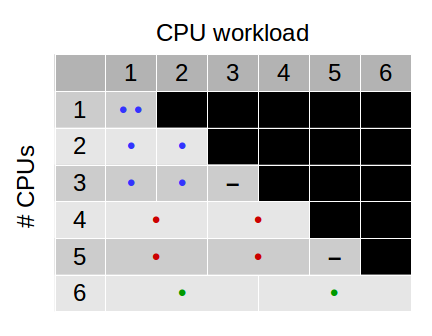Fig. 208 Matrix illustrating how QuantumATK distributes the workload in the case of a bulk calculation with 2 irreducible k-points.

The matrix shows how QuantumATK will automatically distribute the workload in the case of a bulk calculation with 2 irreducible k-points. Each filled circle represents a k-point, i.e., one unit-of-work. Blue, red, and green colors indicate that 1, 2, and 3 processors are used per k-point, respectively. The black dashes indicate that one CPU will be idle if the calculation is parallelized over 3 or 5 processors.

### Processes per k-point¶

The matrix in Fig. 208 shows that choosing the number of computing cores, $$N_\mathrm{MPI}$$, to be either 1 or a multiple of the number of k-points, $$N_k$$, leads to a parallelization scheme that utilizes all the allocated cores. With 4, 6, 8, etc. cores, the workload for each k-point is automatically shared by several cores. However, this kind of parallelization can also be selected manually by the user.

The DiagonalizationSolver calculates the density matrix by direct diagonalization of the Hamiltonian matrix, and the solver accepts the parameter processes_per_kpoint, which sets the number of MPI processes assigned to work on each k-point. In the matrix in Fig. 208, processes_per_kpoint is 1, 2, and 3 for blue, red, and green circles, respectively.

While processes_per_kpoint = Automatic is the default, it is easy to set it using the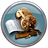Script Generator. Open theCalculator settings window, and select Algorithm Parameters. Make sure DiagonalizationSolver is chosen as eigenvalue solver, and click the Parameters button to open a small window that allows you to manually set the number of processes per k-point.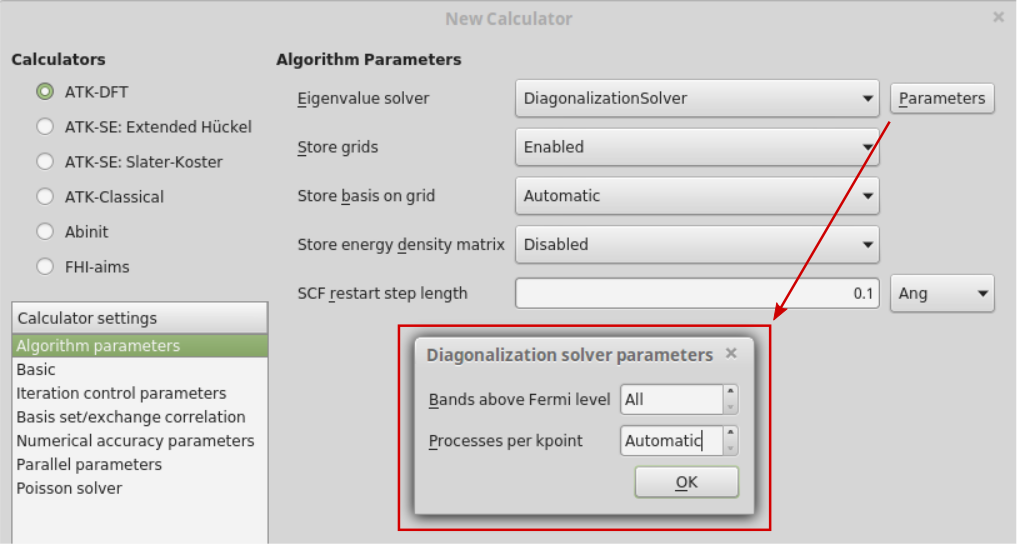### Exercise I: Non-default number of processes per k-point¶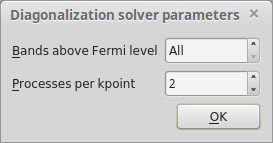Tip

The DiagonalizationSolver also accepts the parameter bands_above_fermi_level, which is by default set to All bands. Explicitly setting this parameter to a positive integer can often be used to speed up the ATK-DFT calculation. However, be aware that too small values of bands_above_fermi_level will lead to inaccuracies in the diagonalization routine.

• Finally, send the script to the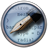Editor to see how the parameter processes_per_kpoint is set in the script. The script will look like shown below.

  1 2 3 4 5 6 7 8 9 10 11 12 13 14 15 16 17 18 19 20 21 22 23 24 25 26 27 28 29 30 31 32 33 34 35 36 37 38 39 40 41 42 43 44 45 46 47 48 49 50 51 52 53 54 55 56 # -*- coding: utf-8 -*- # ------------------------------------------------------------- # Bulk Configuration # ------------------------------------------------------------- # Set up lattice lattice = FaceCenteredCubic(8.1714*Angstrom) # Define elements elements = [Silver, Silver, Silver, Silver, Silver, Silver, Silver, Silver] # Define coordinates fractional_coordinates = [[ 0. , 0. , 0. ], [ 0. , -0. , 0.5], [ 0. , 0.5, -0. ], [ 0. , 0.5, 0.5], [ 0.5, 0. , -0. ], [ 0.5, 0. , 0.5], [ 0.5, 0.5, -0. ], [ 0.5, 0.5, 0.5]] # Set up configuration bulk_configuration = BulkConfiguration( bravais_lattice=lattice, elements=elements, fractional_coordinates=fractional_coordinates ) # ------------------------------------------------------------- # Calculator # ------------------------------------------------------------- k_point_sampling = MonkhorstPackGrid( na=3, nb=3, nc=3, ) numerical_accuracy_parameters = NumericalAccuracyParameters( k_point_sampling=k_point_sampling, ) density_matrix_method = DiagonalizationSolver( processes_per_kpoint=2, ) algorithm_parameters = AlgorithmParameters( density_matrix_method=density_matrix_method, ) calculator = LCAOCalculator( numerical_accuracy_parameters=numerical_accuracy_parameters, algorithm_parameters=algorithm_parameters, ) bulk_configuration.setCalculator(calculator) nlprint(bulk_configuration) bulk_configuration.update() nlsave('Silver.hdf5', bulk_configuration) 
• Notice lines 41–43, where processes_per_kpoint=2 is set for the DiagonalizationSolver. This defines the density matrix method that is passed on to the AlgorithmParameters object, which is eventually given as an option for the LCAOCalculator.

• If you have MPI installed on your machine, you should try to run the calculation with parallelization over 2 cores. Use either the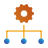Job Manager, or run the script directly from Command-line.\$ mpiexec -n 2 atkpython Silver.py > Silver.log

• In any case, you will the following DiagonalizationSolver parallelization report in the QuantumATK job logfile, here named Silver.log:

+------------------------------------------------------------------------------+
| DiagonalizationSolver parallelization report.                                |
+------------------------------------------------------------------------------+
| Total number of processes: 2                                                 |
| Total number of k-points: 14                                                 |
| Processes per k-point: 2                                                     |
| Number of process groups: 1                                                  |
+------------------------------------------------------------------------------+


### Speed-up and peak memory reduction¶

The figure below illustrates how the calculation wallclock time and peak memory requirement of a simple ATK-DFT calculation may both be reduced by using MPI parallelization. The bulk calculation has 4 irreducible k-points.Fig. 209 Wallclock time and peak memory requirement when parallelizing a simple bulk calculation using MPI.

In Fig. 209, blue triangles indicates wallclock time and red circles indicate the peak memory requirement on each core. Horizontal blue lines indicate 100%, 50%, 25%, and 12.5% of the wallclock time without parallelization (1 MPI process). They indicate perfect efficiency for 1, 2, 4, and 8 MPI processes.

It is clear that a significant speed-up is gained with 2–4 MPI processes, and that using 4 cores (such that each k-point fits onto a single core) reduces the wallclock time by almost a factor of 4. However, 5–7 MPI processes bring no further performance gains, because the extra cores are largely idle (there are only 4 k-points to distribute). A significant speed-up occurs with 8 MPI processes (on 8 cores), where the value of processes_per_kpoint automatically switches from 1 to 2, such that all 8 cores can do useful work. With this in mind, the green circles indicate the most efficient choices for the number of MPI processes.

Regarding peak memory, the most significant memory reduction happens already with 2 MPI processes. The smallest peak memory is obtained with processes_per_kpoint larger than 1, i.e., 8 MPI processes. Bear in mind that the amount of peak memory reduction obtainable by workload distribution is highly system dependent. Large systems will usually offer better opportunities for reducing the memory footprint this way.

## NEGF calculations¶

In a SurfaceConfiguration or in a DeviceConfiguration, the system is effectively treated as infinite along the C cell vector, and as periodic along the A and B cell vectors. The 3D Brillouin zone typical of a BulkConfiguration is thus projected onto a 2D Brillouin zone associated with the periodically repeated system in A and B. The electronic structure of the system is thus solved in a way analogous to a BulkConfiguration in A and B, but in a different way along C.

The GreensFunction method uses a block tridiagonal inversion method used to calculate the Green’s function and lesser Green’s function at each at each $$k$$-point and at each energy $$\varepsilon$$. The lesser Green’s function is then used to obtain the full density matrix of the system $$\hat{D}$$:

$\begin{split}\hat{D} = - \frac{1}{\pi} \int \int \mathrm{Im} G^< (\varepsilon,k)\ d\varepsilon\ dk\end{split}$

where $$G^< (\varepsilon,k)$$ is the lesser Green’s function. In QuantumATK, the meaning of $$\varepsilon$$ depends on whether the system is at equilibrium or out-of-equilibrium:

• Equilibrium : $$\varepsilon$$ is a complex energy and the Green’s function is obtained using complex contour integration
• Non-equilibrium : $$\varepsilon$$ is a real energy and the Green’s function is obtained using real contour integration

In practice, the integration is carried out considering a double weighted sum over two discrete sets of $$k$$-points $$N_k = [k_1,\ldots,k_\mathrm{n}]$$ and energies $$M_\varepsilon = [\varepsilon_1,\ldots,\varepsilon_\mathrm{m}]$$

$\begin{split}\hat{D} = - \frac{1}{\pi} \sum_{i=1}^{N_k} w_{k,i} \sum_{j=1}^{M_\varepsilon} w_{\varepsilon,j} \mathrm{Im} G^< (\varepsilon_j,k_i)\end{split}$

Note

Since the evaluation of the Green’s function at non-equilibrium is done using real contour integration, many more $$\varepsilon$$-points are needed! This also means the calculation can potentially scale to many more processes.

The evaluation of each element $$G^<_{i,j}$$ is the computationally intensive part of the whole procedure and takes approximatively the same time for both i and j, so the double sum can be conveniently rewritten as a single sum over a generalized set of contour points $$N_k + M_\varepsilon = [x_1,\ldots,x_\mathrm{n+m}]$$, which refer both to energies and $$k$$-points:

$\begin{split}\hat{D} = - \frac{1}{\pi} \sum_{i=1}^{N_k + M_\varepsilon} w_{k,\varepsilon,i} \mathrm{Im} G^< (x_i)\end{split}$

Each contour point can be regarded as the unit of work in a NEGF calculation.

### Automatic distribution of contour points¶

By default, QuantumATK tries to distribute the contour points in a NEGF calculation on as many processes as possible. The evaluation of each contour point is done independently, which implies the following distribution rules for $$N_k \times M_\varepsilon$$ contour points:

• $$N_{MPI} = N_k \times M_\varepsilon$$ : An optimal distribution of 1 contour point per process is achieved
• $$N_{MPI} <= N_k \times M_\varepsilon$$: QuantumATK will calculate groups of $$N_{MPI}$$ processes stepwise. If $$N_{MPI}$$ is not a dividend of $$N_k \times M_\varepsilon$$, some processes will be partially idle.
• $$N_{MPI} > N_k \times M_\varepsilon$$ : There will not be any improvement compared to the ideal case, but more memory will be available for each contour point.

### Processes per contour point¶

The option processes_per_contour_point allows to control the number of processes used to solve each individual unit of work. In a device or surface calculation, it can be set in theScript Generator by opening theCalculator settings window, and selecting Device Algorithm Parameters. Make sure GreensFunction is chosen as the method, and click the Parameters button to open a small window that allows you to manually set the number of processes per contour point.The default value processes_per_contour_point = 1 provides the maximum efficiency in the calculation. For very large calculations, setting processes_per_contour_point to a number larger than 1 allows you to increase the available memory per individual unit of work beyond that available for a single process. As an example, suppose you have $$N_k \times M_\varepsilon$$ contour points and $$N_k \times M_\varepsilon$$ processes, each one having a maximum memory available which is only half of that required to solve a contour point. In this case, by setting processes_per_contour_point = 2, two groups of $$(N_k \times M_\varepsilon)/2$$ will be solved in a stepwise fashion by using two cores per unit of work, effectively doubling the memory available per contour point.

### Speedup in a device calculation¶

Fig. 211 shows the parallel scaling of a NEGF calculation of a Ag/Si(100) interface, similar to the one considered in the paper “General atomistic approach for modeling metal–semiconductor interfaces using density functional theory and non-equilibrium Green’s function” [cSMB+16]. The system is calculated at equilibrium using $$N_k$$ = 10 $$k$$-points in the irreducible 2D Brillouin zone, and $$M_\varepsilon$$ = 48 $$\varepsilon$$-points on the complex contour for the evaluation of the equilibrium Green’s function. This results in a total of $$N_k \times M_\varepsilon$$ = 480 contour points.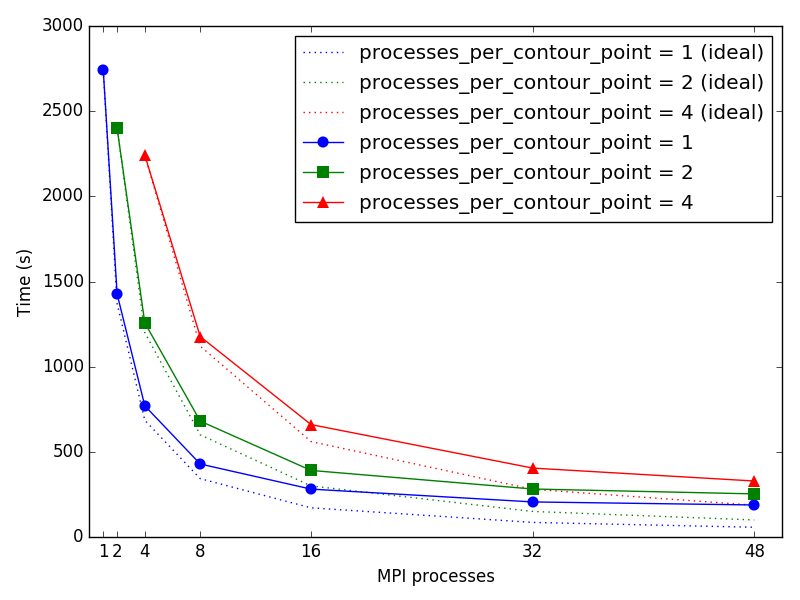Fig. 211 Solid lines: wallclock time when parallelizing a NEGF calculation using MPI, for different values of the option processes_per_contour_point. Dashed lines: ideal scaling of the NEGF calculation based on the time obtained for the minimal number of processes for each value of processes_per_contour_point.

The number of processes $$N_{MPI}$$ has been chosen to be always a divisors of 480, to avoid the presence of idle processes during the calculation. It can be seen that the speedup is close ideal for all the different number of processes considered. As expected, the total walltime for processes_per_contour_point = 2 (processes_per_contour_point = 4) is around two (four) times larger that for processes_per_contour_point = 1 using the same number of processes, because 2 (4) blocks of 240 (120) units of work are solved stepwise. However, the memory available per unit of work is two (four) times larger for processes_per_contour_point = 2 (processes_per_contour_point = 4) compared to processes_per_contour_point = 1.

## Examples of multi-level parallelisms in QuantumATK¶

In the preceding sections, we have discussed the basics of how MPI parallelism can be used to distribute the workload of individual QuantumATK calculations for bulks and devices. However, QuantumATK also offers advanced multi-level parallelism, where the workload of composite calculations may be distributed from the highest level of a hierarchic workflow all the way down to the lowest level, where only one unit-of-work is left.

Such composite calculations include nudged elastic band (NEB) calculations, IV-curve calculations, adaptive kinetic Monte Carlo (AKMC) simulations, and Crystal Structure Prediction using a genetic algorithm, amongst others.

All such methods require a workflow involving calculations for several different structures at the same time, and often quite many of them. Multi-level parallelization makes it possible to run several different calculations for several different structures at the same time, thereby reducing the time-to-result.

Multi-level parallelism is of course automatically enabled if the number of assigned MPI processes is sufficient. However, the Parallel Parameters calculator settings may be used to manually set the desired scheme for multi-level distribution of the workload.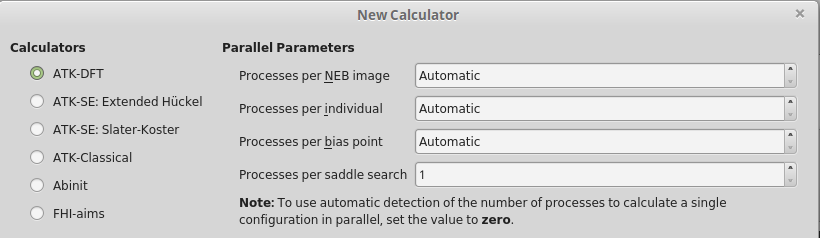### Nudged elastic band calculations¶

Consider first an example of QuantumATK multi-level parallelization of NEB calculations, here using 64 MPI processes in total. The calculation for each of the 4 NEB images has 2 irreducible k-points. The figure below shows how the full NEB calculation is parallelized over first images and then over k-points for each image.• Parallelization level I: 64 CPUs are distributed over 4 groups with 16 CPUs for each group, where each group is assigned to a single NEB image. Setting the number of CPUs per group is automatically done by QuantumATK.

If needed, you may explicitly set the number of MPI processes per NEB image in the QuantumATK Scripter (Processes per NEB image), or set it in the script as follows: parallel_parameters = ParallelParameters(processes_per_neb_image=16).

• Parallelization level II: If the QuantumATK calculator for the NEB calculation uses $$N_{k}$$ k-points for the Brillouin zone integration, e.g., $$N_{k} = 2$$, each image group can be divided into 2 groups with 8 CPUs per k-point. QuantumATK automatically sets this parallelization.

If needed, you may also set the number of MPI processes per k-point manually in the QuantumATK Scripter (New Calculator ‣ Algorithm Parameters ‣ DiagonalizationSolver Parameters).

• Parallelization level III: QuantumATK automatically sets this parallelization.

### I-V curve calculations¶

Computing an I-V curve requires a number of NEGF device calculations at different biases. Multi-level parallelism is therefore used to distribute the workload over bias points and NEGF contour points for each bias.• Parallelization level I: 192 CPUs are distributed over 2 groups with 96 CPUs per group, where each group is assigned to a single bias calculation. Setting the number of CPUs per group is automatically done by QuantumATK.

If needed, you may explicitly set the number of MPI processes per bias point in the QuantumATK Scripter (Processes per bias point), or set it in the script as follows: parallel_parameters = ParallelParameters(processes_per_bias_point=96).

• Parallelization level II: If the QuantumATK calculator for the I-V Curve calculation uses $$N_{e}=48$$ energy points for the NEGF contour integration, and $$N_{k}=2$$ k-points for the 2D Brillouin zone integration at a given energy, each bias group can be divided into 96 groups with 1 CPU per contour (k, energy) point. QuantumATK automatically sets this parallelization.

If needed, you may also set the number of MPI processes per contour point manually in the script as follows: parallel_parameters = ParallelParameters(processes_per_bias_point=96, processes_per_contour_point=1).

### Adaptive Kinetic Monte Carlo simulations¶

The AKMC method is an obvious candidate for multi-level parallelization. A number of saddle searches must be carried out, and QuantumATK will by default try to fully distribute the workload. We will here consider AKMC calculations using ATK-DFT, as efficient parallelization is most important in this case, though AKMC with ATK-ForceField also benefits from parallelization.• Parallelization level I: 192 CPUs are distributed over 48 groups with 4 CPU per group, where each group is assigned to a single saddle point search. Setting the number of CPUs per group is automatically done by QuantumATK.

If needed, you may explicitly set the number of MPI processes per saddle search in the QuantumATK Scripter (Processes per saddle search), or set it in the script as follows: parallel_parameters = ParallelParameters(processes_per_saddle_search=4).

• Parallelization level II: If the QuantumATK calculator for the AKMC calculation uses $$N_{k}$$ k-points for the Brillouin zone integration, e.g., $$N_{k} = 2$$, each image group can be divided into 2 groups with 2 CPUs per k-point. QuantumATK automatically sets this parallelization.

If needed, you may also set the number of MPI processes per k-point manually in the QuantumATK Scripter (New Calculator ‣ Algorithm Parameters ‣ DiagonalizationSolver Parameters).

• Parallelization level III: QuantumATK automatically sets this parallelization.

### Crystal Structure Prediction¶

A genetic algorithm relies on continuously evolving and improving improving generations of candidate minimum-energy configurations. Each generation consists of several (sometimes hundreds or thousands) of different configurations that all need to be geometry optimized. Multi-level parallelism will therefore give a significant speed-up for such calculations in most cases.• Parallelization level I: 192 CPUs are distributed over 48 groups with 4 CPUs per group, where each group is assigned to an individual configuration calculation. Setting the number of CPUs per group is automatically done by QuantumATK.

If needed, you may explicitly set the number of MPI processes per individual configuration in the QuantumATK Scripter (Processes per individual), or set it in the script as follows: parallel_parameters = ParallelParameters(processes_per_individual=4).

• Parallelization level II: If the QuantumATK calculator for the Crystal Structure Prediction calculation uses $$N_{k}$$ k-points for the Brillouin zone integration, e.g., $$N_{k} = 2$$, each image group can be divided into 2 groups with 2 CPUs per k-point. QuantumATK automatically sets this parallelization.

If needed, you may also set the number of MPI processes per k-point manually in the QuantumATK Scripter (New Calculator ‣ Algorithm Parameters ‣ DiagonalizationSolver Parameters).

• Parallelization level III: QuantumATK automatically sets this parallelization.